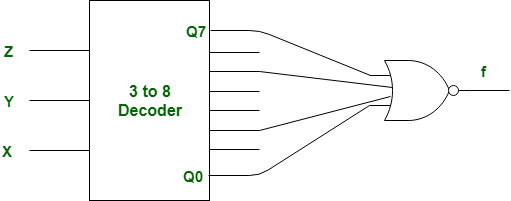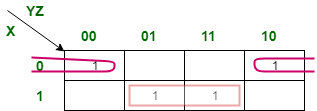# Gate IT 2008

• Last Updated : 02 Nov, 2021

 Question 1
A set of Boolean connectives is functionally complete if all Boolean functions can be synthesized using those. Which of the following sets of connectives is NOT functionally complete?
 A EX-NOR B implication, negation C OR, negation D NAND
Gate IT 2008
Discuss it

Question 1 Explanation:
The EX-NOR is not functionally complete because we cannot synthesize all Boolean functions using EX-NOR gate only. This is primarily because we cannot obtain inverted output using EX-NOR. If we can obtain inversion from any gate then any logic function can be synthesized using that gate only. NAND (A.A)' = A'+A' = A'OR and negation (A+A)' = A'.A' = A'Implication and negation A->B = (A'+B) Now if B is taken as negation of A then A->A' = A'+A' = A' Thus negation can be using these combinations of logics. This solution is contributed by Kriti Kushwaha.
 Question 2
A sample space has two events A and B such that probabilities P(A ∩ B) = 1/2, P(A') = 1/3, P(B') = 1/3. What is P(A U B)?
 A 11/12 B 10/12 C 9/12 D 8/12
Gate IT 2008    Probability
Discuss it

 Question 3
 A 2 B 3 C 4 D 5
Gate IT 2008    Graph Theory
Discuss it

Question 3 Explanation:
The chromatic number of a graph is the smallest number of colors needed to color the vertices so that no two adjacent vertices have the same color. In this graph, minimum number of colors needed to color given graph would be equal to 3.
 Question 4
What is the size of the smallest MIS(Maximal Independent Set) of a chain of nine nodes?
 A 5 B 4 C 3 D 2
Gate IT 2008    Analysis of Algorithms
Discuss it

Question 4 Explanation:
A set of vertices is called independent set such that no two vertices in the set are adjacent. A maximal independent set (MIS) is an independent set which is not subset of any other independent set. The question is about smallest MIS. We can see in below diagram, the three highlighted vertices (2nd, 5th and 8th) form a maximal independent set (not subset of any other MIS) and smallest MIS.
```0----0----0----0----0----0----0----0----0
```
 Question 5
Which of the following regular expressions describes the language over {0, 1} consisting of strings that contain exactly two 1's?
 A (0 + 1) * 11(0 + 1) * B 0 * 110 * C 0 * 10 * 10 * D (0 + 1) * 1(0 + 1) * 1 (0 + 1) *
Gate IT 2008    Regular languages and finite automata
Discuss it

Question 5 Explanation:
By looking at option A and D clearly not feasible solution.
Between B and C both contains exactly two 1's but in option B, both 1 will always come together where as in C it is general string.
 Question 6
Let N be an NFA with n states and let M be the minimized DFA with m states recognizing the same language. Which of the following in NECESSARILY true?
 A m ≤ 2n B n ≤ m C M has one accept state D m = 2n
Gate IT 2008    Regular languages and finite automata
Discuss it

Question 6 Explanation:
A state in a DFA is proper subset of states of NFA of corresponding DFA
=> set of states of NFA =n
=> no of subsets of a set with n elements = 2n
=> m<=2n
 Question 7
The following bit pattern represents a floating point number in IEEE 754 single precision format 1 10000011 101000000000000000000000 The value of the number in decimal form is
 A -10 B -13 C -26 D None of these
Gate IT 2008    Digital Logic & Number representation    Number Representation
Discuss it

Question 7 Explanation:
To convert the floating point into decimal, we have 3 elements in a 32-bit floating point representation: i. Sign ii. Exponent iii. Mantissa Sign bit is the first bit of the binary representation. '1' implies negative number and '0' implies positive number Exponent is decided by the next 8 bits of binary representation. 131-127=4 Hence the exponent of 2 will be 4. i.e 24=16. 127 is the unique number for 32 bit floating point representation. It is known as bias. It is determined by 2k-1-1 where 'k' is the number of bits in exponent field. Thus bias = 3 for 8 bit conversion and 127 for 32 bit. (28-1-1 = 128-1=127) Mantissa is calculated from the remaining 24 bits of the binary representation. It consists of '1' and a fractional part which is determined by: The fractional part of mantissa is given by: 1*(1/2) + 0*(1/4) + 1*(1/8) + 0*(1/16) +.........=0.625 Thus the mantissa will be 1+0.625=1.625 The decimal number hence given as Sign*Exponent*Mantissa = (-1)*(16)*(1.625) = -26. Related : https://www.youtube.com/watch?v=03fhijH6e2w http://quiz.geeksforgeeks.org/number-representation/ This solution is contributed by Kriti Kushwaha.,
 Question 8
Consider the following Boolean function of four variables f(A, B, C, D) = Σ(2, 3, 6, 7, 8, 9, 10, 11, 12, 13) The function is
 A independent of one variable B independent of two variables C independent of three variable D dependent on all the variables
Gate IT 2008
Discuss it

Question 8 Explanation:
The Karnaugh map solutions of the given function can be obtained in two ways. These are the minimal functions that can be obtained. Thus the functions obtained are independent of variable "D".
 A'B' A'B AB AB' 00 01 11 10 A'B' 00 1 1 A'B 01 1 1 AB 11 1 1 AB' 10 1 1 1 1
A'C+AC'+AB'
 A'B' A'B AB AB' 00 0 1 11 10 A'B' 00 1 1 A'B 01 1 1 AB 11 1 1 AB' 10 1 1 1 1
 Question 9
What Boolean function does the circuit below realize ?A xz+x'z' B xz'+x'z C x'y'+yz D xy+y'z'
Gate IT 2008    Digital Logic & Number representation    Combinational Circuits
Discuss it

Question 9 Explanation:
There 0, 2, 5, 7 are enable input in given decoder. Therefore, given K-Map should be as following:So, output of above K-Map is,
```= xz + x'z'
= (x⊙z)
= x (ex-nor) z ```
But, there given gate is NOR instead of OR, therefore above output will be negatated. So, output function f is,
```f = (xz + x'z')'
= x'z + xz'
= (x⊕z)
= x (xor) z ```
So, option (B) is correct. This explanation is contributed by Deep Shah.
 Question 10
Arrange the following functions in increasing asymptotic order:
A. n1/3
B. en
C. n7/4
D. n log9n
E. 1.0000001n
 A A, D, C, E, B B D, A, C, E, B C A, C, D, E, B D A, C, D, B, E
Gate IT 2008    Analysis of Algorithms
Discuss it

Question 10 Explanation:
B and E belong to exponential functions. So {B,E}{A, C, D}. BE. A{C, D}. DC Hence the correct sequence is ADCEB.
There are 82 questions to complete.
My Personal Notes arrow_drop_up